# Estimation of health effects of prenatal methylmercury exposure using structural equation models

## Abstract

### Background

Observational studies in epidemiology always involve concerns regarding validity, especially measurement error, confounding, missing data, and other problems that may affect the study outcomes. Widely used standard statistical techniques, such as multiple regression analysis, may to some extent adjust for these shortcomings. However, structural equations may incorporate most of these considerations, thereby providing overall adjusted estimations of associations. This approach was used in a large epidemiological data set from a prospective study of developmental methyl-mercury toxicity.

### Results

Structural equation models were developed for assessment of the association between biomarkers of prenatal mercury exposure and neuropsychological test scores in 7 year old children. Eleven neurobehavioral outcomes were grouped into motor function and verbally mediated function. Adjustment for local dependence and item bias was necessary for a satisfactory fit of the model, but had little impact on the estimated mercury effects. The mercury effect on the two latent neurobehavioral functions was similar to the strongest effects seen for individual test scores of motor function and verbal skills. Adjustment for contaminant exposure to poly chlorinated biphenyls (PCBs) changed the estimates only marginally, but the mercury effect could be reduced to non-significance by assuming a large measurement error for the PCB biomarker.

### Conclusions

The structural equation analysis allows correction for measurement error in exposure variables, incorporation of multiple outcomes and incomplete cases. This approach therefore deserves to be applied more frequently in the analysis of complex epidemiological data sets.

## Background

Observational studies in epidemiology often involve several sources of uncertainty, such as measurement error, proxy variables of unknown validity, confounder adjustment, and multiple comparisons with outcome variables. Standard statistical methods are poorly suited to deal with these problems, especially when all of them occur at the same time. During the past decade or so, the technique of structural equation analysis has been advanced and made more easily available through software packages. Studies in environmental epidemiology have started to incorporate this approach [1, 2], although most studies have focused on estimating the relative importance of exposure sources, e.g., with regard to lead concentrations in the body . New user-friendly software offers opportunities that we have explored in a complex data set from an environmental epidemiology study on the effects of developmental mercury exposure on nervous system development.

Methylmercury is a common contaminant in seafood and freshwater fish. While adverse effects have been unequivocally demonstrated in poisoning incidents, the implications of lower-level exposures in seafood eating populations have been controversial . Our prospective study of children with developmental methylmercury exposure involved several exposure indicators and several neurobehavioral effect variables assessed at 7 years . These data therefore form a good example of situations where structural equations can be expected to be helpful.

## Materials and methods

### The Faroese Mercury Study

A birth cohort of 1022 children was generated in the Faroe Islands during 1986–1987 and is being studied prospectively to examine the possible adverse effects of developmental exposure to methylmercury. The Faroese population is exposed to methylmercury mainly through consumption of contaminated pilot whale meat. Information about the children's prenatal exposure was obtained mainly by measuring mercury concentrations in biological samples. Two biomarkers of a child's prenatal mercury exposure are available: the mercury concentration in the cord blood (B-Hg) and the maternal hair mercury concentration (H-Hg). Both these exposure measurements are subject to measurement error in the laboratory as well as to biological fluctuations. However, the former biomarker was thought to be the best indicator of the biologically relevant concentration of mercury in the fetal circulation. Additional information about the prenatal mercury exposure was obtained through questionnaire data on maternal diet during pregnancy. Thus, in connection with each birth, a midwife asked the mother about the number of pilot whale dinners per month (Whale).

Because the effects of fetal exposure to methylmercury are likely to be persistent, the children underwent a detailed neuropsychological examination just before school entry, i.e., in 1993–1994, when advanced neurobehavioral testing would be feasible. The children were given neuropsychological tests reflecting different domains of brain function . The tests considered here had virtually complete data for the 917 children examined at age 7 years and did not involve any difficulties in regard to scoring, change of examiner or dependence on postnatal exposure. The tests included are:

• Neurobehavioral Examination System (NES) Finger Tapping: First the child tapped a (computer) key for 15 seconds with preferred hand for practice, then twice with the preferred hand, then twice with the non-preferred hand and finally two keys were tapped with both hands twice. Scores (FT1, FT2 and FT3) are the maximum number of taps under each condition.

• NES Hand Eye Coordination: The child had to follow a sine-wave curve on the computer screen using a joy-stick. The score (HEC) is the average deviation from the stimulus in the best two trails.

• Wechsler Intelligence Scale for Children – Revised Digit Spans: Digit spans of increasing length were presented until the child failed both trials in a series of the same length. The score (DS) is total number of correct trials on the forward condition.

• California Verbal Learning Test (children): A list of 12 words that can be clustered into categories was given over five learning trails, followed by a presentation of an interference list. The child was twice requested to recall the initial list, first immediately after the presentation of the interference list and again 20 minutes later after completing some other tests. Finally, a recognition test was administered. Scores are the total number of correct responses on the learning trials (CV LT1), on the two recall conditions (CV LT2, CV LT3) and on recognition (CV LT4).

• Boston Naming Test: The child was presented with drawings of objects and asked to name the object. If no correct response was produced in 20 seconds a semantic cue was provided describing the type of object represented. If a correct response still was not given, a phonemic cue consisting of the first two letters in the name of the object was presented. The scores are total correct without cues (BNT1) and total correct after cues (BNT2).

### Confounding

A set of confounders was identified by Grandjean et al. , which included sex and age of the child, maternal intelligence (score on Raven's Progressive Matrices) and socio-economic variables. Included in the set of potential confounders is also the child's computer acquaintance. This variable is expected to affect performance on tests performed on the computer but unlikely to be associated with the results on the other tests.

The possibility of confounding in this data set has received much attention . There are two main sources from which confounding may have arisen and which may not have been fully considered in previous analyses.

In addition to methylmercury the Faroese population is exposed to increased levels of polychlorinated biphenyls (PCB). This exposure originates mainly from ingestion of polluted pilot whale blubber. Therefore, exposures to PCB and mercury are positively correlated (after a logarithmic transformation the correlation to the cord blood mercury indicator is 0.40, p < 0.0001). Since PCB is also an established neurotoxicant, the effect of mercury exposure on childhood neurobehavioral ability may be overrated if the effects of PCB exposure are not taken into account. The prenatal PCB exposure was measured as twice the sum of the wet weight concentrations of the three major PCB congeners 138, 153, and 180 in umbilical cord tissue. These congeners are persistent and most reliable as indicators of chronic exposure. However, this information was only obtained from about half of the children (those examined in 1993). In standard analyses only children with complete information on all variables (complete cases) are considered. This is not an optimal solution to this missing data problem, because information about the mercury effect is needlessly lost when attention is restricted to children examined in 1993. Using structural equation models, the mercury effect may first be estimated temporarily ignoring the PCB exposure. A more sophisticated analysis for estimation of the PCB-corrected mercury effect is then developed based on all available information.

Another source from which confounding can arise in this study is that the rural part of the Faroese population tended to eat more fish and whale meat than the residents of the capital of Torshavn (15,000 inhabitants) where the availability of whale meat in 1986–1987 was low. At the same time, capital-living may be associated with predictors of good performance on the neuropsychological tests such as high maternal intelligence and education. A variable (Town7) indicating whether the child was living in one of the three Faroese towns (Torshavn, Klaksvik or Tværå) at the time of the examination is therefore included. This variable has been added to the list of potential confounders mainly because of concern that the rural children perform more poorly, perhaps also in some cases because of fatigue caused by traveling to the test site. In naive multiple regressions (not taking exposure measurement error into account) the urban residents appeared to have an advantage for some outcomes. However, this may be an artifact caused by exposure measurement error and high correlation between the exposure variable and the potential confounder .

### Structural equation modeling

Structural equation models constitute a very general and flexible class of statistical models including ordinary regression models and factor analytic models [7, 8]. The aim is to model the conditional distribution of the observed response variables (y i = (y i,1,...,y i,p )t) given the observed covariates (z i = (z i,1, ..., z i,q )t) of subject i, i = 1,...,n. This is achieved by viewing response variables as indicators of latent variables and by assuming linear regressions between latent variables and covariates.

First, a latent continuous variableis attached to each of the observed response variables. The relation between y i,j anddepends on the nature of the observed variable. For y i,j continuous, one simply lets y i,j =, while a threshold model is postulated if y i,j is ordered categorical with categories 1,2,..., K j

y i,j = k if τ j,k -1≤ τ j,k

where τ j,0 ≤ τ j,1 ≤ ... ≤ τ j,K j are (unknown) thresholds with τ j,0 = -∞ and τ j,K j = ∞.

A structural equation model typically consists of two parts: a measurement model and a structural model. In the measurement model the response variable y i is related to a latent m-dimensional variable η i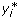= v + Λη i + K z i + ε i ,     (1)

where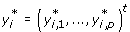, v is a vector of intercepts, Λ is a p × m matrix of so-called factor loadings and ε i is a vector of measurement errors which follow a normal distribution with mean zero and covariance Ω. The matrix K contains regression coefficients which describe direct effects of the covariates on the (latent) response variables. Usually only a few of the rows of K are different from zero.

The structural part of the model describes the relation between the latent variables (η i ) and the covariates

η i = α + Bη i + Γz i + ζ i     (2)

Here α is a vector of intercepts and B is an m × m matrix of regression coefficients describing the relation between the latent variables. The diagonal elements of this matrix is zero and I - B is non-singular. Covariate effects are given by the m × q matrix Γ. Finally, ζ i is an m-dimensional vector of residuals, which is assumed to be independent of the measurement errors ε i , while following a normal distribution with mean zero and variance Ψ.

The model can be extended by letting some parameters depend on a group variable. For example, the parameters of the structural part of the model may depend on the gender of the subject.

### Local dependence and item bias

Under the standard assumptions, response variables are assumed to be independent given the latent construct they are hypothesized to reflect. Local dependence is present when some indicators are correlated beyond the degree explained by the latent construct. For instance, in the analysis of the Faroese data, the test scores FT1, FT2, FT3 and HEC are all assumed to reflect the same latent neurobehavioral function in a child. However, as the three finger tapping scores all originate from the same test protocol, these scores are likely to show additional correlation.

Here local dependence is taken into account by introducing new latent variables which are included in the model as random effects. For example, the finger tapping scores are all assumed to depend on the same latent variable (η5) in addition to the latent neurobehavioral function (η3). To be precise, the measurement part of the model for these outcomes can be expressed as follows: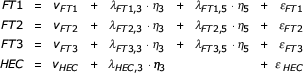Local dependence could also have been introduced by freeing off-diagonal elements in Ω (= var(|z i , η i )). In this way, an excess negative correlation between responses could have been allowed for.

In the measurement model specified above, children at the same level of the latent neurobehavioral function (η3) are expected to have equal test scores on each of the individual tests of that function. If item bias (or differential response function) is present this assumption is violated. A response variable is said to be biased with respect to for instance sex, if boys tend to score consistently higher (or lower) than girls with the same latent ability level.

Item bias is easily incorporated in the model by allowing non-zero parameters in the matrix K (1). Of course, it is not possible to identify item bias with respect to the same covariate for all indicators of a given latent variable. As a minimum one indicator has to be assumed to be unbiased. The choice of the unbiased indicator is not important for the main parameters, i.e., those describing the relation between the latent variables. If another indicator is chosen, then this measurement model is equivalent to the measurement model corresponding to the original choice, expect that the dependence of the latent variable on the covariate responsible for the item bias has changed. However, if the relation between the latent variables is corrected for all covariates in the structural part of the model then the main parameters (B) will remain unchanged. Of course, this does not mean that the same estimates are obtained whether or not a correction for item bias is performed.

### Estimation

The parameters to be estimated are θ = (τ, ν, Λ, K, Ω, α, B, Γ, Ψ) where τ denotes the vector of all unknown thresholds. The likelihood function is derived by noting that the conditional distribution ofgiven z i is N p {μ(θ) + Π(θ)z i , Σ(θ)}, where μ(θ) = ν + Λ (I - B)-1α, Π(θ) = Λ(I-B)-1Γ + K and Σ(θ) = Λ(I - B)-1Ψ (I - B)-1t Λt + Ω. The model is naturally extended by letting μ, Π and Σ vary freely. The resulting model is known as the unrestricted model or the reduced form and plays a central role in the estimation algorithm for θ in models where some response variables are considered categorical.

Assuming independence between subjects the likelihood function becomes L (y,z,θ) =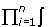Di(| μ(θ) + Π(θ)z i , Σ(θ)) dwhereis the density of the normal distribution, and the i'th domain of integration (D i ) is the set of-values which are mapped onto the observed value of y i .

In models where all response variables are continuous the likelihood function is a product of normal distribution densities, and parameters may be estimated using the maximum likelihood (ML) method. Furthermore, the asymptotic covariance of the ML estimates (ML) can be estimated as the inverse of the expected Fisher information . The ML estimator is consistent even if y i is not normally distributed given z i , but the estimated covariance matrix has to be adjusted. Satorra  provides a sandwich type estimator of the asymptotic covariance ofML, which is robust to the assumption of multivariate normality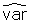(ML) = n -1t C -1Δ)-1 Δt C -1V1 C -1Δ(Δt C -1Δ)-1     (3)

Here C is an estimate of the covariance of the (sufficient) vector consisting of sample means and covariances based on the assumption that (y i , z i ) has an unrestricted normal distribution . The matrix Δ is the derivative ∂σ(θ)/∂θ evaluated atML, where σ is the vector of population means and covariances. Further, V 1 is the asymptotically distribution free (ADF) estimator of the covariance of the sample means and covariances involving fourth-order moments of the data .

In the general case where one or more of the response variables is considered categorical, the likelihood function is an intractable integral possibly of high dimension and ML estimation becomes problematic. Numerical integration methods must be considered, but these methods are computationally demanding and have not yet been incorporated in widely available software for structural equation analysis. Instead, a weighted least squares estimation method suggested by Muthén  may be used. This method is not efficient but it provides estimates that are consistent and asymptotically normally distributed . The method consists of three steps

1. The parameters of the unrestricted model τ, μ, Π and the diagonal elements of Σ are estimated in univariate analyses of each of the p response variables y i,j i = 1, ..., n. Thus, for y i,j continuous the estimates are obtained from an ordinary linear regression model, while an ordinal probit model is fitted if y i,j is ordered categorical. For identification the residual variance of categorical response variables is set to one.

2. The off-diagonal elements of Σ are estimated in the bivariate analyses of all pairs of response variables (y i,j ,y i,k ) i = 1, ..., n. The estimates maximize the likelihood function of the model for the two response variables in the pair given the covariates and the estimates obtained in step 1.

3. The parameters of the unrestricted model are stacked in a vector κ. The parameters of the structural equation model θ are estimated by minimizing a weighted least squares discrepancy

F(θ) = {- κ(θ)}t W -1 {- κ(θ)}     (4)

between the estimated value of κ (obtained in steps 1 and 2) and the parameter values attainable under the structural equation model (κ(θ)). Here W is a weight matrix.

Different choices of weight matrix W are available in user-friendly software. For the so-called WLS (weighted least squares) estimator W = V 2, where V 2 is a consistent estimator of the asymptotic covariance matrix of. The (asymptotic) covariance of this estimator is estimated by evaluating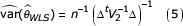at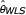, where Δ = ∂κ(θ)/∂θ.

The WLSMV (weighted least squares mean and variance adjusted) estimator uses a diagonal W matrix with estimated variances ofas elements . For this estimator the asymptotic covariance matrix is estimated by(WLSMV ) = n -1t W -1Δ)-1 Δt W -1 V 2 W -1 Δ (Δt W -1 Δ)-1     (6)

Asymptotically, the WLSMV is not as efficient as the WLS estimator. However, in simulation studies, Muthén et al.  found that WLSMV provides a dramatically improved performance compared to WLS. Thus, when sample sizes are moderate, the inclusion of off-diagonal elements in the weight matrix used in WLS estimation seems to introduce noise rather than improve efficiency. Because of this superior performance at moderate sample sizes, the WLSMV estimator is sometimes described as robust .

### Tests of model fit

The fit of a structural equation model is naturally assessed in a two-level process. In the first level, the fit of the unrestricted model as defined above is tested. For models where the response variables are all continuous, a host of well-known model checking techniques are available. For example, normality assumptions and assumptions about variance homogeneity of error terms may be checked from ordinary residual plots, while the linearity of covariate effects can be checked by testing the significance of higher order terms and interaction terms. When categorical responses are present, validation of the unrestricted model is not straightforward, especially if covariates are also included .

In level two, the appropriateness of assumptions of the proposed structural equation model is investigated by testing this model against the unrestricted model. Nested models in which response variables are all continuous are compared using ordinary likelihood ratio testing.

For models where at least one of the response variables is categorical, a large sample χ2-test of model fit (against the unrestricted model) may be obtained as

2·n·F WLS (),     (7)

where F WLS denotes the WLS discrepancy function (4). Accordingly, a large sample test comparing nested models may be obtained noting that the corresponding 2·n·F WLS ()-difference asymptotically has a χ2-distribution with degrees of freedom equal to the difference in dimensions between the models.

Instead of the WLS-test, Muthén et al.  recommended the so-called mean and variance adjusted χ2-test (G MV ), which has better statistical performance when sample sizes are moderate. This statistic is obtained as follows

G MV = {d*/tr(UV 2)}·n·F WLSMV (WLSMV ),     (8)

where U = W -1 - W -1 Δ (Δt W -1Δ)-1) Δt W -1, W is the weight matrix of the WLSMV estimator and d* is the integer closest to {tr(UV 2)}2/tr{(UV 2)2}. This variable is approximately χ2-distributed with d* degrees of freedom. Unfortunately, this statistic cannot be used for comparison of two nested structural equation models since G MV -differences are not χ2-distributed.

Sattora and Bentler originally derived a goodness-of-fit measure similar to (8) for structural equation modeling of continuous non-normal data . They showed that the asymptotic mean and variance of the G MV -statistic are the same as in the described χ2-distribution under very weak distributional assumptions.

The so-called root mean square error of the approximation (RMSEA) offers an alternative method for assessment of goodness-of-fit and is often used in applications of structural equations. This method was developed by Browne and Cudeck  from the point of view that statistical tests of model fit may prevent the use of parsimonious models or large sample sizes. The fit index is calculated as follows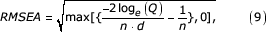where -2 log e (Q) is the likelihood ratio test statistic against the unrestricted model and d is the corresponding number of degrees of freedom. The RMSEA can be considered an estimate of the socalled discrepancy per degree of freedom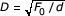, where F 0 = loge (|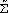0|) - loge (|Σ0|) + tr0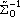) - (p + q) is an (unobservable) measure of the discrepancy between the population covariance matrix (Σ0) of the observed variables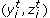and the covariance matrix closest to this in the model (0). Confidence intervals for D can be calculated based on the asymptotic non-central χ2-distribution of -2 log e (Q). A RMSEA-value below 0.05 is considered an indication of a close fit. However, this method is only available for models where all response variables are considered continuous.

### Missing data

Application of the multivariate method described above may introduce a missing data problem. A standard method for handling this problem is to conduct a socalled complete case analysis which is restricted to subjects with no missing values on the variables modeled. However, when many variables (exposures, confounders and responses) are analyzed simultaneously the subset of observations with complete data may be heavily reduced. The Faroese variables have limited missing data problems except for the variable on the child's prenatal PCB exposure which was not measured in approximately half of the children (those examined in 1994). If these values are missing completely at random , a complete case analysis including the PCB variable would yield consistent estimation, but power may be lost. Little and Rubin  described how to perform statistical analysis based on all available information. They showed that under certain assumptions, the missing data mechanism can be ignored and inference can be based solely on the likelihood function of the observed data, which is calculated by integrating out missing values in the likelihood function obtained had data been complete. This method yields consistent estimations under much weaker assumptions than those needed for the complete case analysis.

The theory of Little and Rubin for statistical analysis with missing data is based on the maximum likelihood principle. In structural equation models where some response variables (y) are considered ordinal, the likelihood function is an intractable integral sometimes of high dimension and maximum likelihood estimation is not feasible. Instead least squares methods are used, but these methods are not compatible with important concepts of the missing data theory. However, in the special case where all responses are continuous (and conditionally normally distributed) the likelihood function is simpler, and maximum likelihood estimation can be achieved even when some subjects have missing values.

In structural equation analysis, both response variables and covariates may have missing values. In the following, r denotes the socalled missing data indicator. Thus, r is a vector with dichotomous elements indicating which of the responses and the covariates that are missing for the subject at hand. Here the subject index i has been suppressed for simplicity in notation. Furthermore, (y obs, z obs) denotes the observed variables, while (y mis,z mis) denotes the missing data. For a given subject, the likelihood function of the observed data and the missing data indicator is

L(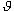, ψ, r, y obs, z obs) = ∫ p ψ (r|y obs, y mis, z obs, z mis) p(y obs, y mis, z obs, z mis) d (y mis, z mis),

where the density p(y obs, y miss, z obs, z mis) denotes the joint density of the responses and the covariates. This density is given as a product of the density from the structural equation model (p θ {y mis,y obs|z obs,z mis}) and the density for the covariates (p ι {z obs, z mis}). Data are said to be missing at random (MAR) if r and (y mis, z mis) are conditionally independent given (y obs, z obs). Thus, under MAR the missing data contain no information about the missing data mechanism beyond what is available through the observed data. Under this assumption, the likelihood function factorizes

L(, ψ, r, y obs, z obs) = p ψ (r|y obs, z obs) ∫ p(y obs, y mis, z obs, z mis) d (y mis, z mis)

If it is further assumed that the two parameter vectorsand ψ are variation independent (distinct), then it is not necessary to model the missing data mechanism, and maximum likelihood inference about θ can be based solely on the likelihood of the observed dataThus, when the covariates have missing values, a model is needed for the distribution of covariates in addition to the structural equation model. The standard solution is to assume that the covariates follow a multivariate normal distribution. However, this assumption is often not appropriate.

In case the covariate information is complete (z obs = z), a model is not needed for the covariates and the likelihood function reduces to

L (θ, y obs, z) = ∫ p θ (y obs, y mis|z) dy mis

The total likelihood function (for all subjects) is obtained as the product of the likelihood functions of each subject (each on the form given above). This function may be maximized using the EM algorithm .

### Path diagrams

When many variables are modeled simultaneously the set of equations defining the structural equation model easily becomes complex. A better understanding of the model assumptions may be provided by a path diagram, which gives a pictorial representation of the model. In a path diagram, observed variables (y i , z i ) are enclosed in boxes while latent variables (ηi,) are in ovals (or circles) with the exception of disturbance terms (ε i , ζ i ). A causal relation is represented by a single-headed arrow from the causal variable to the effect variable. If two variables are connected by a two-headed arrow, this indicates that the variables are correlated but no assumptions about causation are made.

### Software

The data were analyzed using the statistical software packages Mplus, version 1.01 , and MECOSA 3 . In either of these programs most of the statistical methods described above are available. For models consisting of continuous response variables, both programs offer ML estimation and provide standard errors that are robust to the assumption of multivariate normality of residuals. Missing data analysis can be conducted with both programs but for this task the coding is not straightforward in MECOSA 3. In the general case where categorical responses are also present, parameters can be estimated using the WLS method but the more robust WLSMV method is only available in Mplus as is the robust test statistic of model fit G MV (8).

An important difference between the two packages is that model specification is easier in Mplus. While MECOSA 3 requires the user to specify the elements in each of matrices (τ, ν, Λ, K, Ω, α, B, Γ, Ψ) defining the model, models in Mplus are developed by using a number of statements each involving one of three key words. The key word 'BY' is used to relate observed response variables to latent variables in the measurement part of the model. For example, the neuropsychological test scores FT1, FT2, FT3 and HEC are assumed to be error prone indicators of the latent variable η3 using the statement: 'ETA3 BY FT1 FT2 FT3 HEC'. Regression relations (most frequently encountered in the structural part of the model) are described using 'ON'. As an example of this, η3 is assumed to depend linearly on the child's sex and age with the statement: 'ETA3 ON SEX AGE'. Finally, 'WITH' is used to describe correlations both in the measurement part and the structural part of the model. Thus, one way of specifying that the finger tapping scores (FT1, FT2 and FT3) are correlated given η3 (i.e. that these scores are locally dependent) would be to add the three statements: 'FT1 WITH FT2', 'FT1 WITH FT3' and 'FT2 WITH FT3'.

Because Mplus provides robust inferential methods, user-friendly programing and high computational speed, this program was chosen for the final analysis of the Faroese data. In models of continuous response variables, the parameter estimates are obtained using the ML method (default in Mplus). Conventional standard deviation estimates are used (default in Mplus) unless otherwise stated. When ordinal responses are present, the WLSMV estimator is used, and the model fit is assessed by the G MV statistic (8).

## Results

### Model development

Two biomarkers are available in regard to a child's prenatal mercury exposure. After a logarithmic transformation, the relation between mercury concentrations in cord blood and maternal hair is approximately linear. Therefore, the following model for the distribution of the exposure variables appears appropriate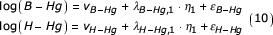where the subject index i has been suppressed for simplicity in notation and the log base is 10. The latent variable η1 represents the true prenatal mercury exposure and is assumed to follow a normal distribution. The model further assumes, that except for measurement error, the two exposure indicators are given as a linear function of the true exposure. Here the measurement error is a sum of two different types of error: laboratory measurement imprecision and biological variation. The second error component arises because the mercury concentration in the fetal circulation is not constant over time but varies according to maternal mercury intake. It may also include individual differences in the distribution of mercury in the body.

The measurement error terms ε B-Hg and ε H-Hg are assumed to be normally distributed with means 0 and variancesand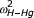, respectively. Furthermore, the blood and hair measurement errors are assumed independent. Methylmercury is thought to have a biological half-life of 45 days or slightly more , so the concentration present in the cord blood reflects the exposure mainly during the last couple of months of gestation. If the active dose is some sort of a long-term average mercury concentration, then the assumption of independence between measurement errors in cord blood and in maternal hair may be appropriate, because digested mercury is deposited in the hair with a lag time of up to 6 weeks. This lag-time may ensure that the two biomarkers are not affected by the same random biological fluctuations on a temporal scale. In addition, concentrations of mercury in hair and in cord blood were determined by two different laboratories  which means that analytical errors are unlikely to be correlated.

For identifiability the cord blood factor loading is fixed at one (λ B-Hg = 1), thus the true mercury exposure has the same scale as the (log-transformed) cord blood concentration. The mean of η1 is identified by fixing the intercet ν B-Hg at zero. However, even with these restrictions, there are too many free parameters and the exposure part of model is not identified. Additional information on the prenatal mercury exposure is available from the questionnaire data on maternal pilot whale meat consumption during pregnancy. The distribution of the ordered categorical variable Whale (5 categories: 0,1,2,3,≥ 4) is modeled introducing a latent continuous variable (Whale*) and assuming a threshold relation. In this example, the continuous latent variable could represent the weight of ingested whale meat.

Intake of pilot whale meat differs fundamentally from the measurements of mercury concentrations in hair and blood. While the latter two are determined (with a certain measurement error) by the true exposure (η1), it may seem more natural to consider pilot whale meat intake as a determinant of a true exposure: an increase in maternal whale meat intake will increase the mercury exposure, not the other way around. Bollen  describes such response variables as cause indicators as opposed to the two biomarkers which enter the model as effect indicators. From (2) it is seen that latent variables can only be affected by covariates and other latent variables. Thus, to incorporate this cause indicator in the current modeling framework formally it is necessary to introduce an additional latent variable η2. This latent variable is identical to Whale* and assumed to affect the latent mercury exposure. With three indicators of the latent variable η1, the exposure part of the model is identified.

For the neuropsychological test scores, the optimal structural equation analysis would assume a single latent outcome variable. However, with tests that spanned from computerized assessment of motor speed to delayed recall of nouns, the scores considered clearly depend on different functional domains. The effect variables were therefore sorted into major nervous system functions, with one group consisting of motor functions, the other group encompassing cognitive function with a verbal component. Thus, it is assumed that the scores on the NES-tests (FT1, FT2, FT3 and HEC) are all indicators of an underlying motor function (η3), while the scores on BNT, the CVLT and Digit Spans are all indicators of a latent verbally mediated function (η4). Although this categorization may appear as a severe simplification of diverse outcomes that may depend on multiple functional domains, this analytical approach may be reasonable given the multifocal or diffuse effects of mercury neurotoxicity. To define the scales of the two latent neurobehavioral functions, the factor loadings of the responses FT1 and BNT2 are fixed at one. In agreement with previous analyses performed by Grandjean et al. [5, 23], the neuropsychological outcome variables are all modeled as continuous (conditionally) normally distributed variables. As a starting point, the elements of the measurement error vector (ε i ) are assumed independent.

The true mercury exposure is hypothesized to affect the two latent outcome functions negatively after adjustment for effects of covariates. Thus, the structural part of the model is η = α + Bη + Γz + ζ with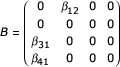where β31 and β41 denote the effect of mercury exposure on the motor function and the verbally mediated function, respectively. These parameters indicate the effect of prenatal mercury exposure corrected for measurement error, and they constitute the parameters of main interest in this analysis.

Maternal whale meat intake is assumed to affect the child's mercury exposure, but no direct effects of Whale* (= η2) on the cognitive functions are present in the model (β32 = β42 = 0). In other words, the true mercury exposure is considered an intermediate variable in the relation between maternal whale meat intake and the child's neurobehavioral function.

Potential confounders of the association between mercury exposure and child test performance are included in the model as covariates. By constraining the appropriate Γ-coefficients (2) to zero, it is assumed that computer acquaintance has no effect on the verbally mediated test scores. None of the exposure-confounder associations are ruled out in advance. Thus, the means of the latent variables η1 and Whale* are assumed to depend linearly on each of the confounders.

The first component of the disturbance term ζ = (ζ1, ..., ζ4)t models the conditional distribution of the true mercury exposure given the covariates and intake of whale meat. The second component describes the conditional distribution of Whale* given the covariates. The last two components give the conditional distribution of the two latent neurobehavioral functions given the covariates and the latent mercury exposure. These two components are not assumed to be independent as motor and verbal functioning are expected to be positively correlated given the values on covariates and true mercury exposure. Figure 1 gives the path diagram illustrating the initial model for these data.

### Correction for local dependence and item bias

Unfortunately, the proposed model does not fit the data adequately when compared to the unrestricted model (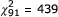, p < 0.0001). The correlation structure assumed for the neurobehavioral test scores is clearly too simple. The assumption that a child's test scores are independent given the latent level on the neurobehavioral functions is violated here, because the eleven outcomes originate from only five separate test protocols. Scores from the same test protocol are likely to show local dependence.

Local dependence is now modeled introducing three new latent variables (η567), which enter the model as random effects. In addition to the latent motor function, the finger tapping tests (FT1, FT2 and FT3) are all assumed to depend linearly on η5, which is normally distributed with zero mean and independent of all other variables. This random effect can be interpreted as indicating how good the child is at the common task, key tapping, corrected for the more general motor ability. In the same way η6 and η7 describe local dependence for the BNT-scores and the CVLT-scores, respectively. The path diagram in Figure 2 illustrates how local dependence between indicators of neurobehavioral functions is incorporated in the structural equation model.

As expected, none of the three random effects could be ignored: in (naive) Wald tests random effect variances are highly significant with u-statistics between 5.11 (CVLT) and 6.59 (FT). Furthermore, all random effect factor loadings are highly significant (data not shown). Incorporation of local dependence improves the model fit substantially, but the fit of the unrestricted model is still significantly better (, p < 0.0001).

In this analysis, a consequence of the assumption of no item bias is that the covariates are assumed to affect indicators of the same latent cognitive function in the same way except for scale differences. For example, the ratio between mercury corrected regression coefficients of a given covariate on the first two finger tapping tests is equal to the ratio of the motor function factor loadings (λ FT1,3 FT2,3). Comparisons of regression coefficients obtained in naive multiple regressions for each indicator suggested that the assumption of no item bias is not satisfied for the study outcomes.

Here item bias is identified successively for the covariates. For a given covariate, item bias parameters are included for all indicators expect FT1 and CV LT1, which are chosen as the reference outcomes. Parameters that are insignificant in successive tests (backward elimination) are removed from the model and a new covariate is considered the same way. The covariates are analyzed in the order indicated by Table 4, starting with covariates a priori thought to be most important (i.e., the child's age and sex and maternal intelligence). To avoid identification of spurious effects using this multiple testing procedure, only parameters with a numeric u-statistic above 2.5 were considered significant.

The extended model with six item bias parameters gives a very good fit (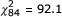, p = 0.26). Despite the strong improvement in model fit, the estimated mercury effects (Table 1) changed only slightly as a result of correction for local dependence and item bias. Thus, for the motor function it is estimated that the effect of a tenfold increase in the true mercury exposure corresponded to a loss of about 1 point on the finger tapping test with preferred hand (FT1). For the verbally mediated function the effect a similar exposure increase corresponded to a loss of about 1.6 points on the cued BNT-score (BNT2). The latter effect is highly significant with a p-value below 0.002 while the motor effect is on verge of statistical significance using the conventional level of 5%.

In Mplus it is not possible to obtain a mean and variance corrected test (8) of the overall hypothesis of the no mercury effect (β31 = β41 = 0). Differences between mean and variance corrected test statistics may not follow a χ2-distribution. However, exploiting that the estimators (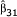and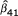) asymptotically follow a normal distribution, an ordinary Wald statistic can be calculated for this hypothesis. The correlation between the two mercury effect estimators was estimated at 0.098, which means that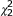= 13.33 with p = 0.0013. Using the WLS fit statistic (7) the hypothesis of no mercury effect can be tested directly. This test yielded a-value of 31.13 corresponding to a p-value below 1/106. Thus, the overall test was clearly more statistically significant using WLS inference. Simulation studies have shown that the WLSMV has better statistical properties than the WLS . Therefore, the test based on the WLSMV estimates probably yielded the most reliable result. This was confirmed in later analyses using maximum likelihood inference.

Table 2 shows estimated factor loadings (λ) and measurement error variances (ω2) for the two biomarkers of prenatal mercury exposure. These estimates show very little variation across the models considered, therefore only the estimates of the model including adjustments for local dependence and item bias are given. The quality of an indicator is not determined solely by the measurement error variance. When indicators have different factor loadings the measurement error variances are on different scales and cannot be directly compared. The indicator with the largest error variance might be the best indicator if it also has the largest factor loading. The measurement error standard deviation of the maternal hair concentration was therefore converted to the scale of the cord blood concentration after multiplication by the absolute value of the factor loading ratio (ω H-Hg ·|λ B-Hg H-Hg |). From the converted error variances (Table 2), it is seen that the cord blood mercury gives the most precise reflection of the true exposure. This result is in agreement with a priori expectations and with the results of Grandjean et al. [5, 23] showing that in multiple regressions the cord blood concentration generally was a stronger predictor of childhood cognitive deficits than the maternal hair concentration. However, the error variance of the cord blood indicator, corresponds to a coefficient of variation of 28%. This result is approximately four times the documented analytical imprecision .

After local dependence has been taken into account, the variance of most indicators are assumed to come from three different sources of variation: variation explained by the latent neurobehavioral function, variation due to the random effect of the test subgroup, and indicator specific variation. For each indicator, Table 3 shows how the total variance is distributed on these three variance components. Thus, the first column of the table gives the percentage of the total variation explained by the latent neurobehavioral function, i.e., the so-called reliability ratio . From these data it is seen that the neurobehavioral indicators generally are noisy with relatively low values between 10.4% to 66.0%. The two BNT scores measure the verbally mediated function with the greatest precision. For the CVLT-scores, reliability ratios decrease from learning to delayed recall and recognition. The Digit Span test measures the verbally mediated ability level of a given child with the same precision as short-delay recall on the CVLT-test. According to the model, the CVLT recognition test (CV LT4) is a poor indicator of verbal ability. Another possibility is of course that this test does not measure the same brain function as the other CVLT-scores. This explanation may also be appropriate for the motor indicator HEC which has a reliability ratio about half that of the finger tapping tests.

The last column of Table 3 illustrates how the definition of the latent neurobehavioral functions has changed after taking local dependence into account. For each test score, the ratio (in percent) between the reliability ratios with and without correction for local dependence is given. If this ratio is above 100%, then the indicator at hand measures the latent function with greater precision as a result of the correction for local dependence. This is seen to be the case especially for HEC and DS, which is not surprising. Both scores are alone in their subgroup. More weight is placed on such variables in the definition of the latent variables when extra correlation between the other indicators of the same latent variable is taken into account. The relative changes in reliability ratio may seem dramatic, but it should be noted that inclusion of local dependence changed the estimated mercury effects only slightly. Furthermore, effects of covariates (data not shown) also changed very little as a result of the correction for local dependence.

Six significant item bias parameters were identified (Table 4). Four of these parameters regard item bias caused by the child's sex, i.e., that the relation between test scores in boys and girls differed between tests reflecting the same neurobehavioral function. In the original approach, where FT1 was chosen as the (unbiased) reference outcome, none of the parameters describing item bias of the child's sex could be removed for the motor indicators. However, the ratio of the bias parameters of FT2 and FT3 corresponded closely to the ratio of motor factor loadings (λ FT2,3 FT3,3). Thus, if FT2 (and not FT1) was chosen as the unbiased estimator then the coefficient of FT3 was clearly insignificant. This more parsimonious representation was therefore preferred in the final analysis. For the two BNT scores, which are on approximately the same scale (λ BNT1,4 = 0.993, λ BNT2,4 = 1), item bias of almost the same size was identified for the child's sex. For these outcomes another way to introduce item bias is to let the mean of the random effect (η7) depend on the child's sex. In this way item bias is introduced on a test-subgroup level using only one parameter.

Table 4 shows the estimated effects of the covariates on the two latent neurobehavioral functions as well as the direct covariate effects on the biased indicators. As before, all regression coefficients of motor responses are on the scale of the FT1-test, while all regression coefficients of verbal responses are on the scale of BNT2. At the time of examination the ages of the Faroese children spanned from 6.3 years to 8.2 years, and age is a strong predictor of a good test performance. The relation between achievement levels of boys and girls varied for the motor outcomes. The general trend (as expressed by FT2 and FT3) was that boys did better than girls (2.06 FT1 points). However, for FT1 the advantage of being a boy was significantly smaller (2.06 - 1.43 = 0.64 FT1 points), while girls had an advantage on the HEC error score. Variation in sex effects were also seen for the verbally mediated tests. Here girls generally performed slightly better than boys, but on DS the girls clearly got better results, while boys were better on the BNT. The mother's intelligence, i.e., her score on the Raven test, was a strong predictor of good verbal functioning, but predicted motor outcomes rather weakly, except for the scores on HEC. Presence of major medical risk factors for neurobehavioral dysfunction was negatively associated with neurobehavioral functioning. Children in day care had a strong advantage on the verbal tests, while a slight disadvantage was seen on the motor tests. Vocational or professional education of each parent and the employment status of the father were weakly associated with motor ability. Stronger positive effects of these variables were seen for the verbal outcomes, but only the effect of paternal education was significant at the 5% level. For CV LT2 (short-term recall), paternal employment status was a very strong predictor. For both latent neurobehavioral functions the child's residence at the time of the examination was on the verge of being significant, indicating that urban children did slightly better than rural children. Finally, as expected, a strong positive effect was seen of computer acquaintance on the performance on the computer assisted tests.

### ML estimation, missing data analysis and PCB correction

The aim of the following analysis is to estimate the mercury effect after correction for the effects of prenatal exposure to PCB. Unfortunately, for about half of the children no biomarker information is available on the PCB exposure. In standard analysis only children with complete information on all variables (complete cases) are considered. This is not an optimal solution, because information about the mercury effect is needlessly lost when attention is restricted to children with a PCB value. In Mplus it is possible to conduct an analysis, which takes into account also the incomplete cases, and which yields consistent estimation under the weaker assumption that data are missing at random. Before the PCB variable is included, a missing data analysis is performed to investigate the appropriateness of the underlying assumption of the previous complete case analysis that data are missing completely at random.

Mplus only allows missing data analysis in models where all response variables can be considered to be continuous and normally distributed given the covariates. In the structural equation model developed, only the variable on the maternal whale meat intake is considered ordinal. After a transformation (t(x) = log(x + 1)) this variable is approximately linearly associated with the cord blood mercury concentrations (the best indicator of true exposure). A model where all response variables are continuous was then obtained by replacing the original ordinal variable by the transformed counterpart.

This multivariate normal model fitted the data adequately. The likelihood ratio test against the unrestricted model yielded a p-value of around 1% and an RMSEA (9) of 1.9% with an upper 90% confidence limit of 2.6%. Furthermore, parameter estimates changed only slightly as a result of replacing the ordinal variable and changing the estimation method from weighted least squares (WLSMV) to maximum likelihood (ML). Table 1 shows ML estimates of the mercury effects on the two latent neurobehavioral functions. It is also noticed that the estimated standard deviations of the ML estimates were slightly higher than the standard deviations of WLSMV estimates. This finding may seem a little surprising because the WLSMV is expected to be less efficient. With the WLS method, estimated standard deviations (data not shown) were even lower than with WLSMV, thus again indicating that inference based on this method may be too optimistic. This observation is further supported by the overall test of no mercury effects. In the continuous model, the likelihood ratio test statistic was 13.61, which when evaluated in a-distribution yielded a p-value of 0.0011. This result is in good agreement with the overall test based on WLSMV statistics (= 13.33 with p = 0.0013), but clearly not as significant as the possibly exaggerated WLS result given above (= 31.13 with p < 1/106).

As already mentioned, when the covariates have missing values, a model is needed for the distribution of covariates in addition to the structural equation model. The standard solution in Mplus is to assume that the covariates follow a multivariate normal distribution. However, this assumption is not appropriate in the current data where most covariates are dichotomous. For the variables considered so far (i.e., disregarding the PCB exposure), 706 of 917 children constitute complete cases. Of the incomplete cases, 71 children have missing covariate information. However, the variable on the maternal Raven score is clearly the largest source. If this variable is disregarded only 14 children have incomplete covariate information. To avoid unreasonable model assumptions these 14 children are excluded in the following analysis. Thus, the remaining 903 children have complete covariate information except for the maternal Raven score. However, an ordinary multiple regression analysis revealed that, given the other covariates, the scores on the Raven test with good approximation can be assumed to follow a normal distribution. To obtain a data set without missing values on the covariates, the maternal Raven score was therefore removed from the set of covariates to the set of response variables. This was done without changing the structure of the relations between the maternal Raven score and the other variables and under the assumption that this response variable was measured without error.

Table 1 also gives the estimated parameters of the structural equation after including children with incomplete information. It is seen that these are not markedly different from the estimates of the complete case analysis, indicating that data are missing completely at random. The estimated adverse effect of mercury exposure on verbal functioning dropped to the level of the weighted least squares analysis, while the estimated effect on the motor function became slightly stronger. As expected, the estimated standard errors of the estimates decreased after taking (almost) all available information into account. As a consequence, both mercury effects reached statistical significance at the 5% level.

At this point the PCB exposure was included in the model in place of the mercury exposure. Because the PCB exposure indicator has missing values it cannot be included without making distributional assumptions. After a logarithmic transformation, complete case regression analysis indicated that the PCB exposures are approximately normally distributed (given the covariates) with a linear relation to the neuropsychological test scores. Thus, the PCB exposure entered the model as a response variable, assumed to be affected by the covariates as well as maternal intake of whale meat. Because the measurement error in the PCB variable is not taken into account here, the estimated PCB effects may be biased low (numerically), but the significance tests are likely to be valid.

From Table 5 it is seen that the estimated PCB effect on the motor function was very weak if at all present. The PCB effect on the verbally mediated function was stronger and just significant at the 5%-level. This result is in good agreement with the results obtained using ordinary complete case multiple regression analysis without correcting for the mercury effect . For the neuropsychological tests considered here, this standard analysis showed significant (p < 0.10) PCB effects only for the two BNT-scores (BNT1, BNT2).

The estimated effects of mercury and PCB may be compared using standardized coefficients. For the verbally mediated function, the standardized effect estimate of the PCB exposure was -0.10. Thus, if the PCB exposure is increased by one standard deviation then this cognitive ability is decreased by 0.10 standard deviations. For the mercury exposure the corresponding number was -0.14. The standardized effect on the motor function was -0.01 for PCB and -0.11 for mercury. It should be noted that only the mercury effects was corrected for measurement error.

Indicators of mercury and PCB were then included in the same structural equation model to allow estimation of the individual effect of both exposures. The two sets of indicators entered the model as in the separate analyses, taking into account that exposure to PCB and mercury may be correlated given the confounders and the variable on maternal whale meat intake. While the PCB exposure was first assumed to be measured without error, this assumption is clearly not realistic. Still the long half-life of PCB congeners as compared to that for methylmercury should lead to an exposure indicator less sensitive to short-term fluctuations in maternal marine food intake. However, normal analytical imprecision could easily be 10% (coefficient of variation), to which some biological variation would be added.

When estimating the mercury effect adjusted for possible effects of PCB exposure it is important to take the imprecision in the PCB marker into account. As a result of the strong correlation between exposure levels to mercury and PCB, failure to correct for PCB measurement error can lead to de-attenuated estimates of the mercury effect [6, 21]. Only one biomarker of PCB exposure is available, which means the total measurement error in this indicator cannot be identified in the structural equation analysis. Instead, the significance of PCB measurement error for inference on the mercury effect was investigated in sensitivity analyses assuming different values for the PCB measurement error variance (Table 6). The marginal variances of the PCB concentrations (log transformed) and the cord blood mercury concentrations (log transformed) are approximately equal, so the two exposure indicators have about the same reliability ratio if the log10(PCB) measurement error variance is assumed to be 0.02 (i.e. a coefficient of variation of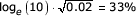on PCB). If instead a log10(PCB) measurement error variance of 0.04 is assumed, then the reliability ratio of the PCB exposure indicator is about the same as that of the maternal hair mercury concentrations. Figure 3 shows the path diagram of the structural part of the model including exposures to both mercury and PCB.

Perhaps somewhat unexpectedly, it is seen from Table 6 that the mercury regression coefficient on motor function was de-attenuated when adjusted for the effect of prenatal PCB exposure. This may indicate that the model was not strong enough to allow simultaneous analysis of these correlated exposures. On the other hand the mercury coefficient was still significant, which would typically not be the case in situations with multicollinearity problems. Residual confounding represents an alternative explanation of the de-attenuated mercury coefficient. When the size of the PCB measurement error was increased the estimated adverse mercury effect increased further, but at the same time it also became less significant.

For the verbally mediated function the mercury-corrected PCB effect was strongly attenuated and far from being statistically significant no matter how large the PCB measurement error was assumed to be. However, as expected, the PCB coefficient was negative, and the mercury effect was attenuated after the PCB correction. This attenuation became stronger the larger the PCB measurement error variance was assumed to be, and the mercury p-value was also sensitive to assumptions about the PCB measurement error. Thus, the mercury effect was significant (5% level) when the PCB indicator was assumed to be error free, but it became insignificant assuming that the error coefficient of variation in the PCB measurement was 46%. The same tendency was seen in the overall test for no mercury effects. In all analyses, the PCB effect remained far from significant.

### Validation of the unrestricted model

So far, the models considered have been tested only against the unrestricted model, but the assumptions of this larger model should also be checked. The ordinal exposure indicator Whale was replaced by a continuous variable, with only minimal changes in the main results. Thus, the appropriateness of the unrestricted model where all response variables are continuous was therefore considered. Residual plots (not shown) indicated that, given the covariates the distributions of most responses were approximately normal. One indicator (CV LT4) deviated from normality with too many children achieving the maximum score. However, the main results did not change when this variable was excluded. Furthermore, the robustness of the inference on the mercury effect to the assumption of multivariate normality was investigated by calculating robust standard deviations (3) for the ML estimates. This approach yielded standard deviations of 0.571 and 0.514 for the mercury effect on the motor function and the verbally mediated function, respectively. These standard deviations are calculated in a complete case analysis, and should therefore be comparable to the standard deviations given in Table 1 (ML estimation after full adjustment). The robust standard deviations are very similar to the ones obtained using normal distribution theory, indicating that the main result of this analysis is robust to the assumption of multivariate normality.

In addition to assumptions about multivariate normality of residuals, the unrestricted model assumes that the observed variables are linearly related. The appropriateness of the logarithmic dose response model for the effect of the two mercury biomarkers on the neurobehavioral outcomes has been carefully investigated using standard multiple regression methods (Budtz-Jørgensen et al., 1999, unpublished results). Likewise, regression analyses failed to identify significant differences of mercury effects in boys and girls . The strong effect of the child's age on the neurobehavioral test scores was investigated by including higher order terms. No important deviations from linearity were found.

### The influence of mercury exposure on the definition of the neurobehavioral functions

In the models considered above, the parameters defining the latent variables are estimated simultaneously in joint analyses of all indicators. Using this approach the mercury exposure indicators may affect the measurement parameters of the two neurobehavioral functions. In other words the meaning of the latent constructs 'motor' and 'verbal' may depend on the exposure variables in addition to the neurobehavioral indicators. The risk that this influence is substantial may be reduced by the fact that all models considered are identifiable even if the exposure variables are disregarded.

The influence of the exposure indicators on the definition of latent neurobehavioral functions and vice versa may be investigated as follows. Two separate analyses were performed based on the multivariate normal model with adjustment for local dependence and item bias. First the parameters were estimated after exclusion of the exposure variables. Then the model was fitted again, this time disregarding the neurobehavioral indicators. In this way two sets of parameters were obtained in which the exposure indicators could not affect neurobehavioral parameters and vice versa. Finally, the model was fitted to all indicators fixing the factor loadings (Λ), the residual variances (Ω) and the parameter describing the effect of pilot whale intake on mercury exposure (β12) at the values obtained from the separate analyses. The variances of the latent variables incorporating local dependence were also fixed, but the residual variances of latent exposure and the latent neurobehavioral functions were kept free. Covariate effects were not fixed since their interpretation depends on whether they are corrected for the exposure effect. The result of the analysis with fixed parameters and the corresponding analysis without parameter constraints are given in Table 7. It is seen that the estimated mercury effects are only slightly attenuated in the fixed analysis, indicating that latent neurobehavioral functions are defined almost entirely by the neurobehavioral indicators, and that the latent exposure variable likewise is virtually unaffected by the outcome parameters.

### Standard analysis

As a final consideration, the results of the structural equation analysis not corrected for the PCB effect are compared to the results obtained using standard multiple regression analysis. Table 8 shows estimated mercury effects obtained in complete case multiple regression analysis for the two main indicators of the exposure. These results differ from those previously published  because the covariate Town7 has been added to the set of potential confounders. The cord blood coefficient of the indicators FT1 and BNT2 are on the same scale as β31 and β41, respectively, of the structural equation models. It is seen that the two sets of parameters are approximately equal. Since the parameters of the structural equation model are corrected for measurement error in the exposure variables it may seem a little surprising that these coefficients are not numerically larger than the naive regression coefficients. This attenuation is caused by the introduction of the latent neurobehavioral functions that take into regard several test results. In a structural equation model with a latent exposure, but with no assumptions on the covariance matrix of the residuals of the neurobehavioral outcome variables, the estimated coefficients corresponded closely to the naive regression coefficients corrected for the estimated amount of measurement error in the exposure variables (data not shown).

A serious weakness of the standard analysis is that the result is quite complex. Table 8 contains 22 regression coefficients each on its own scale. Some coefficients are seen to be highly significant while others are clearly not. With 22 tests of the hypothesis of no mercury effect it is not surprising that some coefficients are significant. Thus, although the regression coefficients all suggest that the exposure is associated with a neurobehavioral deficit, it is not immediately clear form the standard analysis output whether or not the mercury effect is 'overall' statistically significant. For each of the exposure indicators, an overall test of the mercury effect may be obtained in a multivariate regression model assuming that the residuals of indicators are normally distributed with an unrestricted covariance matrix. The significance of the mercury effect is then assessed by testing the hypothesis that the mercury coefficient is zero for all outcome variables. This test was significant with a p-value of 2.45% for the cord blood indicator, while the test yielded a p-value of 9.70% for the maternal hair indicator. For comparison, in the structural equation analysis, the overall test was clearly significant with a p-value of 0.13%. Thus, in addition to providing a simpler presentation of the results, the structural equation approach yielded a stronger analysis.

## Discussion

Observational studies in epidemiology always involve concerns regarding validity, especially measurement error, confounding, missing data, and other problems that may affect the study outcomes. Widely used standard statistical techniques, such as multiple regression analysis, may to some extent adjust for these shortcomings. However, structural equations may incorporate most of these considerations, thereby providing overall adjusted estimations of associations. In environmental epidemiology studies, this technique has especially been used to determine the importance of various sources of lead exposure as reflected in lead concentrations in blood or bone [1, 3].

Although user-friendly software is now available, fitting structural equation models to the observed data may entail several complex steps. In the present study, limited distributional problems were resolved using transformations. In addition, subjects with missing data were included in the analysis under the assumption that data were missing at random. Likewise, intermediate response variables were inserted, e.g., to allow inclusion of the dietary questionnaire response as a predictor of the latent exposure variable. Further, item bias and local dependence were resolved using procedures included as routine functions in the software. By taking these considerations into account a model with a nice fit was obtained, and the estimated exposure effects remained stable. The choice of method for parameter estimation and tests of model fit required the recent method known as WLSMV to be used. In agreement with recent findings  the traditional WLS method yielded test statistics and p-values that seem overly optimistic.

In environmental epidemiological studies, it is usually impossible to obtain an error-free measurement of the exposure. It is well known that if measurement error in the exposure variable is ignored then estimation of the effects of the exposure may be biased . In this case, the cord blood mercury concentration had been considered the most appropriate measure of the fetal mercury exposure . The maternal hair mercury concentration measure may be affected by hair color, hair treatment and other parameters that do not increase the variability of the cord blood concentration . The latent exposure variable is highly useful in this situation, where more than one exposure variable is available each being associated with an unknown imprecision. While taking into regard possible imprecision originating from both analytical error and from biological variation, the maximum information is retrieved from the data. The results of the structural equation analysis show that the cord blood is also the most precise from a statistical viewpoint. Considering the toxicokinetic issues, this result is entirely coherent. The effect estimates provided by this analysis take into regard also the supplementary information available in the hair concentration levels, and they also include adjustments for imprecision in the exposure assessment.

In this regard, it may be noted that the latent exposure variable did not change when taking into account the response information. This finding suggests that the model is not necessarily calibrated according to the strongest exposure-response associations. In the present study, the strong associations between the exposure predictors determined the definition of the latent exposure variable. In contrast, the associations with the outcome variables were rather weak and therefore influenced the latent exposure variable only minimally. This observation means that all outcome variables in the model are forced to relate to the same latent exposure variable. In regard to developmental methylmercury exposure, we have previously discussed that the temporal windows of susceptibility may differ between different domains of brain function . For example, motor function may be more vulnerable to exposures earlier in gestation than the verbally mediated functions. Such notions may not be possible to explore with the structural equation models presented in this paper.

Using structural equation modeling, outcome variables can be grouped in one or more categories, thus providing an overall evaluation of an exposure effect on the total outcome. This approach avoids multiple comparisons, but it exploits all available information without reductions to scales. In this analysis, outcomes were collected in two groups based on a priori knowledge. The initial model fitted data rather poorly, but after corrections for local dependence and item bias, a structural model with a close fit and virtually unchanged exposure effect estimates was obtained. This model thereby yielded a simple representation of the main trends in the complex data set. Thus, a strong mercury effect was identified for verbally mediated outcomes, while a weaker mercury-related deficit was seen for motor outcomes.

Accordingly, the heterogeneity of the response parameters was resolved by creating two different latent response variables, as confirmed by the excellent fit of the model. The strength of the structural equation approach requires that outcomes be grouped in this way, although it may violate neuropsychological notions of separate functional domains being involved in the clinical tests administered. In this regard, it is noteworthy that the definition of the latent response variables did not depend on the inclusion of the mercury exposure variable, an indication that the psycho-metrically most valid tests played the main role, while the much weaker association with mercury exposure only marginally affected the definition of the latent response variables. Thus, because of the design of the model and the properties of structural equation analysis, this analysis does not provide any evidence whether developmental methylmercury exposure has a particular profile, except that mercury seems to affect verbally mediated functions more than motor functions. Also, since the latent response variable was optimized in accordance with the associations with the individual neuropsychological tests, this analysis assumed that all children were affected the same way, i.e., it ignored that the children could theoretically differ in regard to vulnerability of functional domains. However, the strength of the model developed would argue against such variability being an important consideration in this data set.

Structural equations are especially valuable when many endpoints are modeled jointly. However, in epidemiological data sets this multivariate approach is likely to introduce a missing data problem. If all outcomes are considered continuous, maximum likelihood estimation is feasible, and the problem can then sometimes be solved by adopting the approach of Little and Rubin . When some outcomes are modeled as ordinal, estimation is restricted to weighted least squares methods, thus limiting the analysis to the complete cases. For epidemiological applications, structural equation modeling would become even more attractive if user-friendly methods were developed for obtaining maximum likelihood estimates in models with ordinal outcomes. Since the weighted least squares methods are not efficient, this approach may also improve the statistical inference for complete data.

The analysis of PCB effects illustrates the difficulty of separating the effects of two correlated exposures both measured with error and where error adjustment is conducted by different approaches. The marginal analyses show that there is without much doubt an effect of at least one of the exposures. Further, there is almost enough information in the data to rule out that the observed mercury effect for fixed PCB exposure level has arisen by chance. Only if it is assumed that the PCB indicator is very imprecise (error coefficient of variation of about 46% or more) can the observed mercury effect be dismissed as a chance finding (at the conventional level of 5%). Available data on the quality of the PCB analysis suggest that the error is unlikely to be large . On the other hand, based on these data, it cannot be ruled out that prenatal exposure to PCB has no effect for fixed levels of the mercury exposure. In separate analyses using stratification , PCB appeared to show stronger associations with the outcome variables in the tertile group of children with the highest mercury exposure. This potential interaction was not taken into account in the present analysis.

The Faroese mercury toxicity study is a highly appropriate example of a complex data base where extensive structural equation modeling may be helpful. Because of the societal importance of developmental neurotoxicity caused by prenatal exposure to methylmercury, expert groups  have critically reviewed the data and suggested further statistical analyses to explore the possible significance of potential weaknesses of the study. Current risk assessment is based on mercury effects on single outcome tests . The present paper addresses these concerns, thereby illustrating that the structural equations may provide a highly useful supplementary approach. Of particular interest, the overall mercury effect is quite similar to the strongest mercury effects identified in multiple regression analyses of motor and verbal functions. This finding supports the notion that, in this study, the multiple regression findings are valid and that the various sources of error do not seriously impact on the study validity. However, such agreement between different statistical approaches is by no means guaranteed, and structural equations therefore deserve to be considered for independent analyses.

Regulatory agencies have increasingly relied upon calculation of benchmark dose levels from dose-effect relationship. Thus, in the absence of a clear-cut threshold level, the data are used to calculate a lower confidence limit of the dose that leads to a specified increased risk of an abnormal response. In regard to methylmercury, the benchmark dose has been calculated for various data sets as a basis for developing exposure limits . Given the advantages of structural equation analysis, we suggest that benchmark dose calculations should also consider the dose-effect relationships obtained in structural equation analysis of complex data sets.

## References

1. 1.

Buncher CR, Succop PA, Dietrich KN: Structural equation modeling in environmental health assessment. Environmental Health Perspectives. 1990, 107: 481-487.

2. 2.

Vreugdenhil HJI, Duivenvoorden HJ, Weisglas-Kuperus N: The relative importance of prenatal PCB exposure, feeding type, and parental characteristics for cognitive and motor development in healthy children studied from 3 to 84 months of age. Organohalogen Compounds. 2000, 48: 139-142.

3. 3.

Lanphear BP, Roghmann KJ: Pathways of lead exposure in urban children. Environmental Research. 1997, 74: 67-73. 10.1006/enrs.1997.3726.

4. 4.

National Academy of Sciences: Toxicological Effects of Methylmercury. National Academy Press. 2000

5. 5.

Grandjean P, Weihe P, White RF, Debes F, Araki S, Yokoyama K, Murata K, Sørensen N, Dahl R, Jørgensen PJ: Cognitive deficit in 7-year-old children with prenatal exposure to methylmercury. Neurotoxicology and Teratology. 1997, 19: 417-428. 10.1016/S0892-0362(97)00097-4.

6. 6.

Budtz-Jørgensen E, Keiding N, Grandjean P, Weihe P, White RF: Consequences of Exposure Measurement Error for Confounder Identification in Environmental Epidemiology. Research Report 01/13, Department of Biostatistics, University of Copenhagen. 2001

7. 7.

Bollen KA: Structural Equations with Latent Variables. John Wiley and Sons,. 1989

8. 8.

Arminger G, Wittenberg J, Schepers A: MECOSA 3 User Guide. Friedrichsdorf, Germay: ADDITIVE GmbH. 1996

9. 9.

Jöreskog KG: A general method for estimating a linear structural equation system. In Structural Equation Models in the Social Sciences. Edited by: Goldberger AS, Duncan OS. 1973, New York, Seminar Press, 85-112.

10. 10.

Arminger G, Schoenberg RJ: Pseudo maximum likelihood estimation and a test for misspecification in mean and covariance structure models. Psychometrika. 1989, 54: 409-425.

11. 11.

Satorra A: Asymptotic robust in the analysis of mean and covariance structures. In: Sociological Methodology. Edited by: Marsden PV. 1992, Oxford, England, Blackwell Publishers, 249-278.

12. 12.

Muthén B: Latent variable modeling in heterogeneous populations. Psychometrika. 1989, 54: 557-585.

13. 13.

Muthén B: A general structural model with dichotomous, ordered categorical and continuous latent variable indicators. Psychometrika. 1984, 49: 115-132.

14. 14.

Muthén B, du Toit SHC, Spisic D: Robust inference using weighted least squares and quadratic estimating equations in latent variable modeling with categorical and continuous outcomes. Psychometrika. 1997, [http://www.statmodel.com]

15. 15.

Muthén LK, Muthén B: Mplus. The Comprehensive Modeling Program for Applied Researchers. User's Guide. Los Angeles, Muthén & Muthén. 1998

16. 16.

Muthén B: Goodness of fit with categorical and other non-normal variables. In: Testing Structural Equation Models. Edited by: Bollen KA, Long JS. 1993, Newbury Park, CA Sage, 205-234.

17. 17.

Satorra A, Bentler PM: Corrections to test statistics and standard errors in covariance structure analysis. In: Latent Variable Analysis: Applications to Developmental Research. Edited by: von Eye A, Clogg CC. 1994, Newbury Park, Sage Publications, 399-419.

18. 18.

Browne MW, Cudeck R: Alternative ways of assessing model fit. In: Testing Structural Equation Models. Edited by: Bollen K, Long JS. 1993, Newbury Park, Sage Publications, 136-162.

19. 19.

Little RJA, Rubin DB: Statistical Analysis With Missing Data. Wiley. 1987

20. 20.

Grandjean P, Weihe P, Bruse VW, Needham LL, Storr-Hansen E, Heinzow B, Debes F, Murata K, Simonsen H, Ellefsen P, Budtz-Jørgensen E, Keiding N, White RF: Neurobehavioral deficits associated with PCB in 7-year-old children with prenatally exposed to neurotoxicants. Neurotoxicology and Teratology. 2001, 23: 305-317. 10.1016/S0892-0362(01)00155-6.

21. 21.

Carroll RJ, Ruppert D, Stefanski LA: Measurement Error in Nonlinear Models. New York, Chapman and Hall. 1995

22. 22.

Grandjean P, Weihe P, Jørgensen PJ, Clarkson T, Cernichiari E, Viderø T: Impact of maternal seafood diet on fetal exposure to mercury, selenium, and lead. Archives of Environmental Health. 1992, 47: 185-195.

23. 23.

Grandjean P, Budtz-Jørgensen E, White RF, Jørgensen PJ, Weihe P, Debes F, Keiding N: Methylmercury exposure biomarkers as indicators of neurotoxicity in children aged 7 years. American Journal of Epidemiology. 1999, 150: 301-305.

24. 24.

Fuller WA: Measurement Error Models. New York, Wiley. 1987

25. 25.

Grandjean P, Jørgensen PJ, Weihe P: Validity of mercury exposure biomarkers. In: Biomarkers of Environmentally Associated Disease. Edited by: Wilson SH, Suk WA. Boca Raton, FL, CRC Press/Lewis Publishers

### Pre-publication history

1. The pre-publication history for this paper can be accessed here:http://0-www.biomedcentral.com.brum.beds.ac.uk/content/backmatter/1476-069X-1-2-b1.pdf

## Acknowledgements

This study was supported by grants from the National Institute of Environmental Health Sciences (ES06112 and ES09797), the U.S. Environmental Protection Agency (9W-0262-NAEX), the European Commission (Environment Research Programme), the Danish Medical Research Council, and the Danish Health Insurance Foundation. The contents of this paper are solely the responsibility of the authors and do not necessarily represent the official views of the NIEHS, NIH or any other funding agency.

## Author information

Correspondence to Esben Budtz-Jørgensen.

### Authors' contributions

PG and PW designed the cohort study, EBJ, PG and NK developed the strategy for the statistical analysis, EBJ carried out the analyses and wrote the report, and all authors contributed to the data interpretation and the final version of the paper.

None declared.

## Authors’ original submitted files for images

Below are the links to the authors’ original submitted files for images.

## Rights and permissions

Reprints and Permissions

Budtz-Jørgensen, E., Keiding, N., Grandjean, P. et al. Estimation of health effects of prenatal methylmercury exposure using structural equation models. Environ Health 1, 2 (2002) doi:10.1186/1476-069X-1-2

• #### DOI

https://doi.org/10.1186/1476-069X-1-2

### Keywords

• Mercury Exposure
• Local Dependence
• Unrestricted Model
• Item Bias
• Measurement Error Variance